Question1. According to Pauling rule for the pk1 value, the compounds having more oxo groups have less pk1 value and will be the strong acid in nature.

pKa= (8-5p), where P is the number of oxo groups attach to the central metal atom.

Therefore,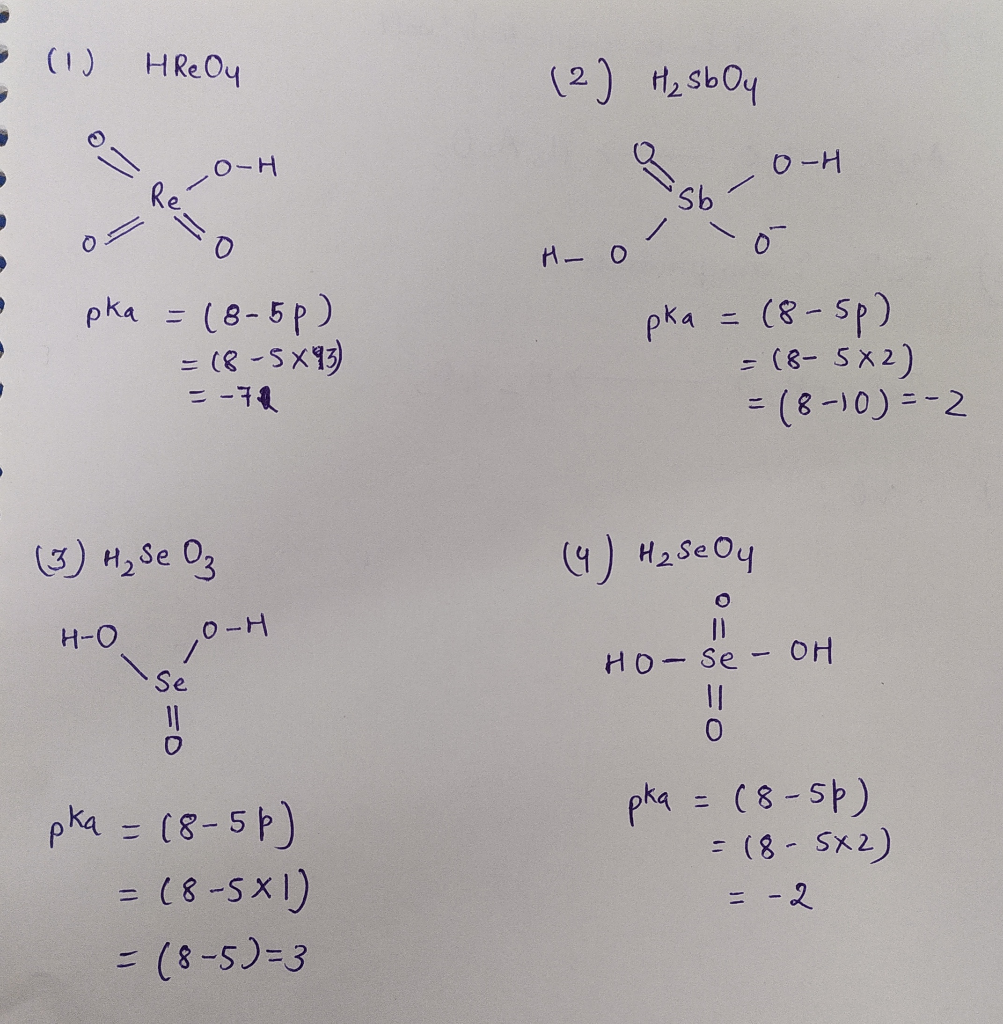The order of acid strength is

1>2>4>3

b.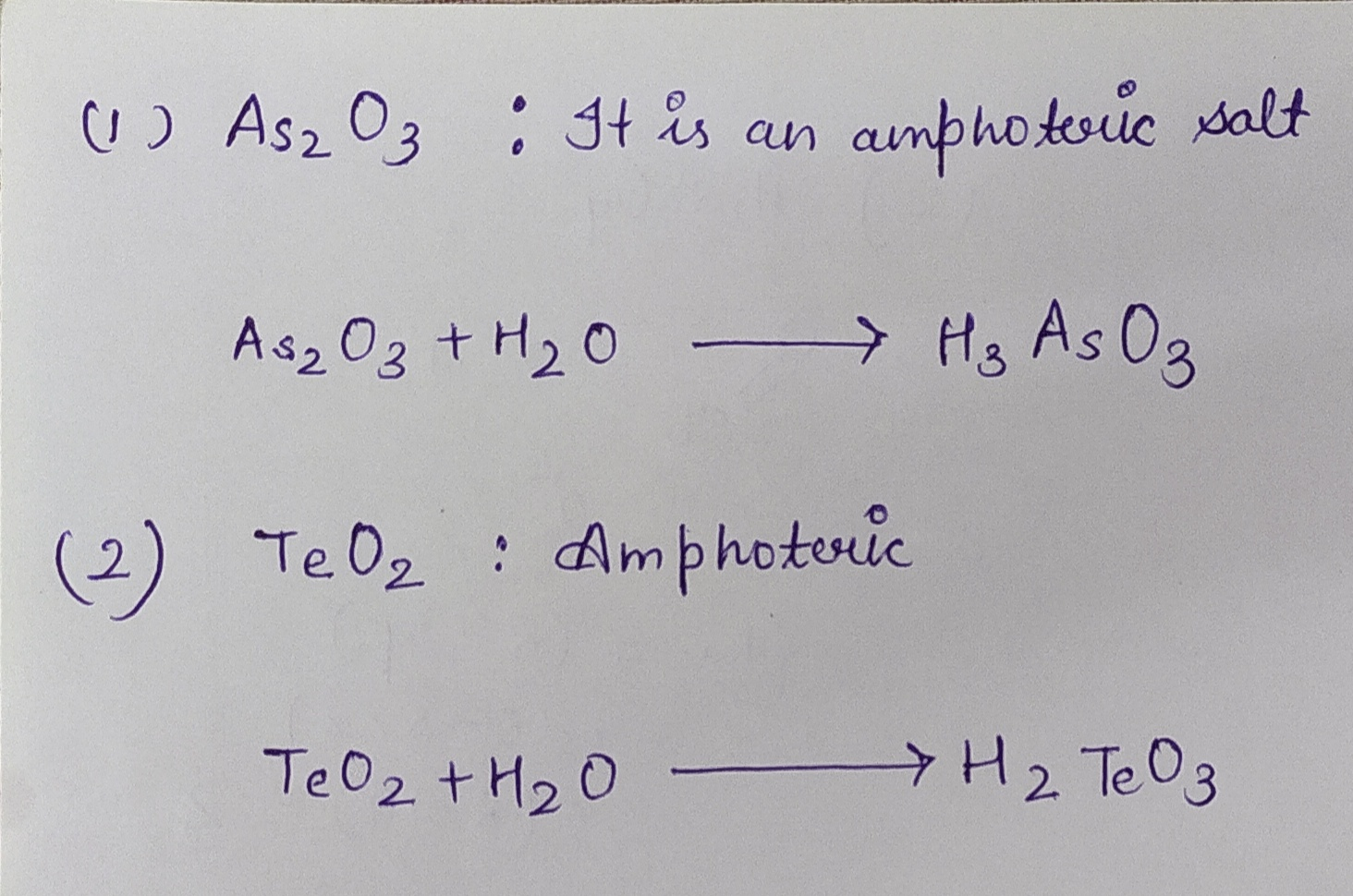#### Earn Coins

Coins can be redeemed for fabulous gifts.

Similar Homework Help Questions
• ### Due. Ilurs. Nov. 1, 2019 1. a. Using Pauling's Rules estimate the pKj values for each...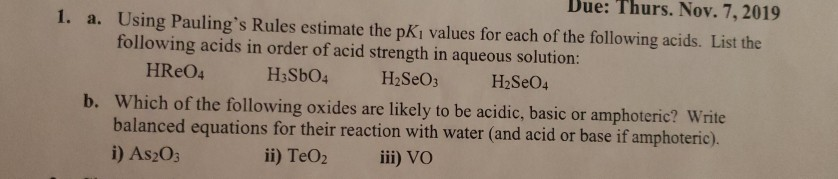Due. Ilurs. Nov. 1, 2019 1. a. Using Pauling's Rules estimate the pKj values for each of the following acids. List the following acids in order of acid strength in aqueous solution: HReO4 HzSbO4 H2SO3 H2SO4 b. Which of the following oxides are likely to be acidic, basic or amphoteric? Write balanced equations for their reaction with water and acid or base if amphoteric). i) As2O3 ii) TeO2 iii) VO

• ### please answer all parts of this question and explain everything 5. Using HSAB theory, choose the...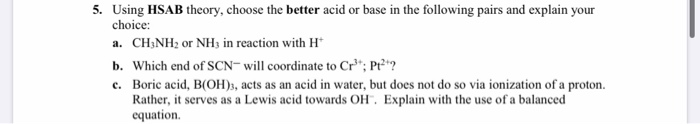please answer all parts of this question and explain everything 5. Using HSAB theory, choose the better acid or base in the following pairs and explain your choice: a. CH3NH2 or NH3 in reaction with H b. Which end of SCN will coordinate to Cr"; Pt?? c. Boric acid, B(OH)3, acts as an acid in water, but does not do so via ionization of a proton. Rather, it serves as a Lewis acid towards OH. Explain with the use of...

• ### please answer all parts of this question thank you 4. Consider each of the following solvents individually: i) NH2CH...please answer all parts of this question thank you 4. Consider each of the following solvents individually: i) NH2CH3 ii) CF3COOH iii) H2SO4. a. Give the equation for the autoionization of the pure solvent. b. Discuss what will happen if H20 is dissolved in each of the solvents, i.e., what ions will form. Give appropriate equations. Will the solution be acidic or basic with respect to the pure solvent? Will the solute act as a weak or a strong acid...

• ### General Acid-Base Concepts – Answer the following questions or provide explanations for the following using any...

General Acid-Base Concepts – Answer the following questions or provide explanations for the following using any and all of the acid-base concepts discussed in Chapter 6. (3 Pts each = 18 Pts) a. The conductivity of BrF3 is increased by adding KF. b. AlF3 is insoluble in HF but dissolves if NaF is added. However, if BF3 is added AlF3 precipitates. c. Most of the metals used in antiquity were class (b) (soft in HSAB terminology) metals. (Hint: look up...

• ### please answer all parts of this question and explain everything 3. For each of the following...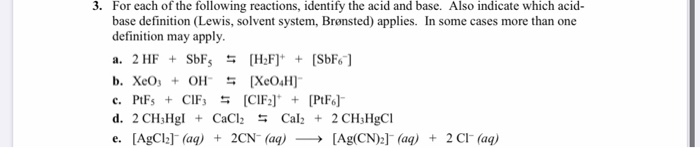please answer all parts of this question and explain everything 3. For each of the following reactions, identify the acid and base. Also indicate which acid- base definition (Lewis, solvent system, Brønsted) applies. In some cases more than one definition may apply. a. 2 HF + SbFs 5 [H2F] + [SbF6] b. XeO; + OH = [XO H) c. PtFs + CIF3 = (CIF)* + [PtF6] d. 2 CH3Hg. + CaCl2 = Cal2 + 2 CH3HgCI e. [AgCl2] (aq) +...

• ### please answer all parts part 1 part 2 (c) part 3 Designate the Bronsted-Lowry acid and...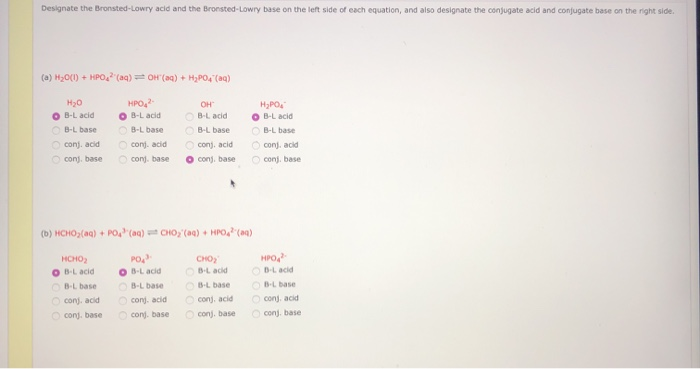please answer all parts part 1 part 2 (c) part 3 Designate the Bronsted-Lowry acid and the Bronsted-Lowry base on the left side of each equation, and also designate the conjugate acid and conjugate base on the right side. (a) H2O(0) + HPO 2(aq) = OH(aq) + H2PO4 (aq) H2O OB-L acid B-L base conj. acid conj. base HPO, 2- OB-Lacid B-L base conj. acid con base он B-L add B-L base conj, acid conj, base НРО B-L acid B-L...

• ### **PLEASE ANSWER ALL QUESTIONS AND EXPLAIN ** TUTORIAL 7. GROUP 16 ELEMENTS 1. What are the...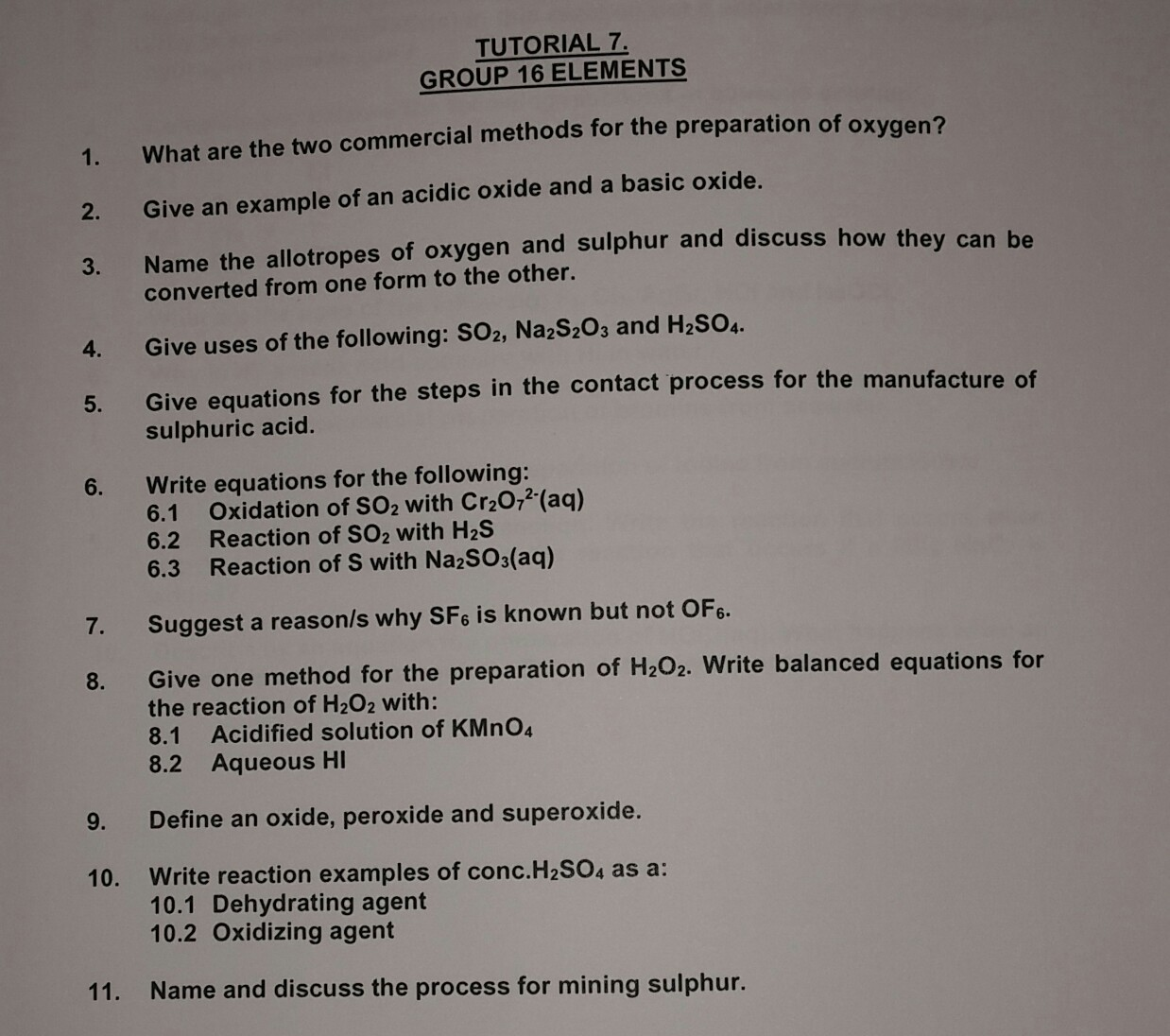**PLEASE ANSWER ALL QUESTIONS AND EXPLAIN ** TUTORIAL 7. GROUP 16 ELEMENTS 1. What are the two commercial methods for the preparation of oxygen? 2. Give an example of an acidic oxide and a basic oxide. 3. Name the allotropes of oxygen and sulphur and discuss how they can he 4. Give uses of the following: SO2, Na2S20s and H2SO4. 5. Give equations for the steps in the contact process for the manufacture o 6. Write equations for the following:...

• ### Help with all questions please Answer all questions on a separate sheet of paper. Show all...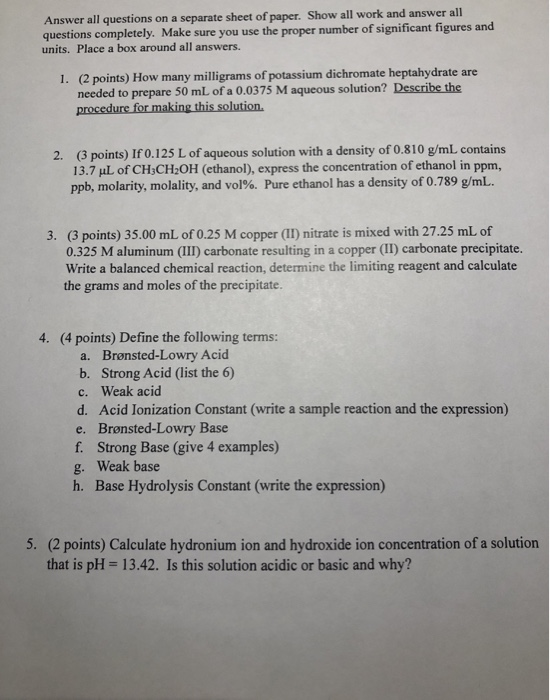Help with all questions please Answer all questions on a separate sheet of paper. Show all work and answer all questions completely. Make sure you use the proper number of significant figures and units. Place a box around all answers. 1. (2 points) How many milligrams of potassium dichromate heptahydrate are needed to prepare 50 mL of a 0.0375 M aqueous solution? Describe the procedure for making this solution. 2. (3 points) If 0.125 L of aqueous solution with a...

• ### Please answer question B parts 1-7 RELAB 4 Reactions in Aqueous Solutions Worksheet A A. Write...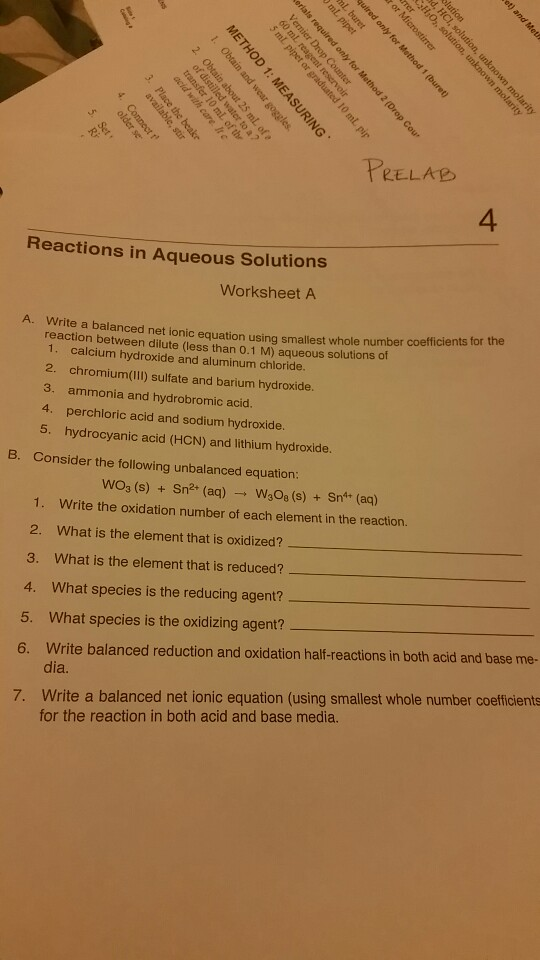Please answer question B parts 1-7 RELAB 4 Reactions in Aqueous Solutions Worksheet A A. Write a balanced net i reaction between dilute (less than 0.1 M) aqueous solutions of onic equation using smallest whole number coefficients for the 1. 2, 3. 4. 5. calcium hydroxide and aluminum chloride. chromium(III) sulfate and barium hydroxide ammonia and hydrobromic acid perchloric acid and sodium hydroxide hydrocyanic acid (HCN) and lithium hydroxide. B. Consider the following unbalanced equation: WO3 (s) + Sn2+ (aq)...

• ### please write legible. answer all questions. 1. Identify each of the following reactions as a precipitation...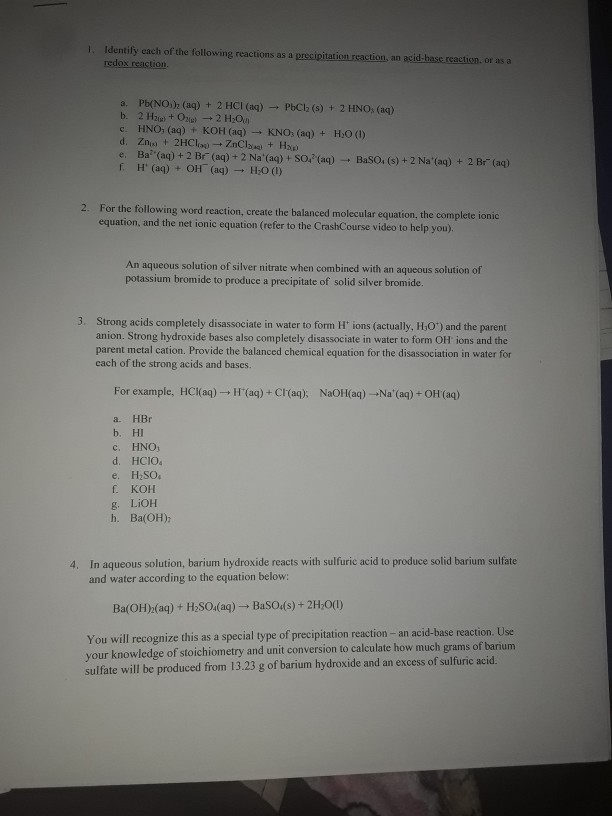please write legible. answer all questions. 1. Identify each of the following reactions as a precipitation reaction, an acid-base reaction or as a redox reaction Pb(NO), (aq) + 2 HCl(aq) - PbCl(s) + 2 HNO(aq) b. 2 H2 + Oxel - 2 H2O CHNO, (aq) + KOH(aq) - KNO, (aq) + H2O (1) d. Zn + 2HCl - ZnCl + H e. Ba"(aq) + 2 Br(aq) + 2 Na' (aq) +SO. (aq) BaSO. (s) + 2 Na'(aq) + 2 Br"...